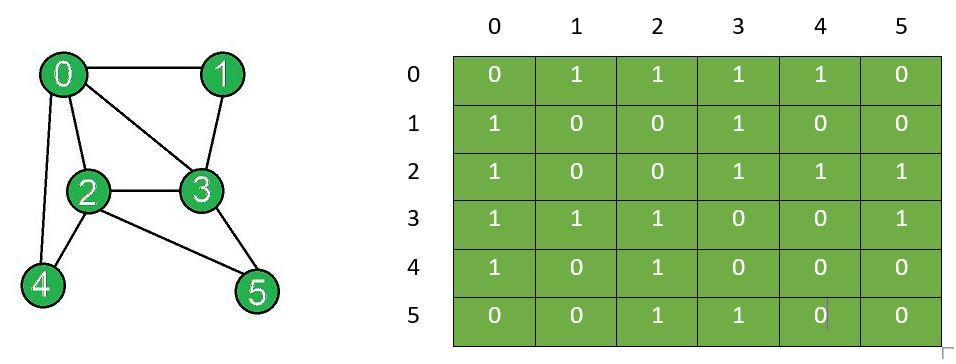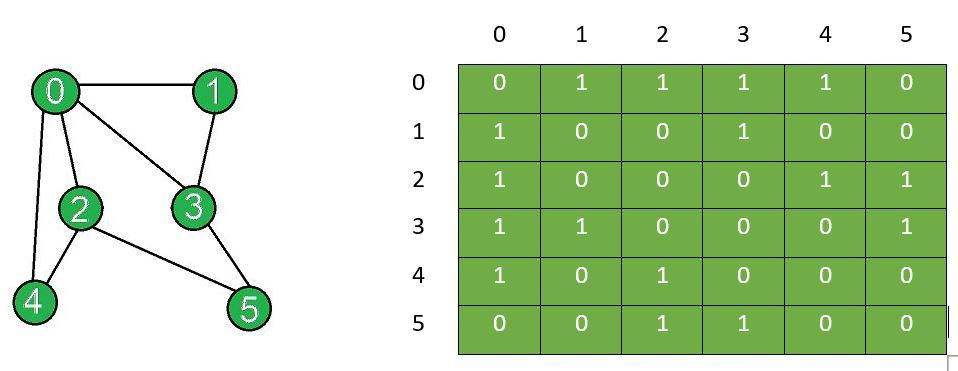# Add and Remove Edge in Adjacency Matrix representation of a Graph

Prerequisites: Graph and its representations

Given an adjacency matrix g[][] of a graph consisting of N vertices, the task is to modify the matrix after insertion of all edges[] and removal of edge between vertices (X, Y). In an adjacency matrix, if an edge exists between vertices i and j of the graph, then g[i][j] = 1 and g[j][i] = 1. If no edge exists between these two vertices, then g[i][j] = 0 and g[j][i] = 0.

Examples:

Input: N = 6, Edges[] = {{0, 1}, {0, 2}, {0, 3}, {0, 4}, {1, 3}, {2, 3}, {2, 4}, {2, 5}, {3, 5}}, X = 2, Y = 3
Output:

0 1 1 1 1 0
1 0 0 1 0 0
1 0 0 1 1 1
1 1 1 0 0 1
1 0 1 0 0 0
0 0 1 1 0 0

0 1 1 1 1 0
1 0 0 1 0 0
1 0 0 0 1 1
1 1 0 0 0 1
1 0 1 0 0 0
0 0 1 1 0 0
Explanation:
The graph and the corresponding adjacency matrix after insertion of edges:The graph after removal and adjacency matrix after removal of edge between vertex X and Y:Input: N = 6, Edges[] = {{0, 1}, {0, 2}, {0, 3}, {0, 4}, {1, 3}, {2, 3}, {2, 4}, {2, 5}, {3, 5}}, X = 3, Y = 5
Output:

0 1 1 1 1 0
1 0 0 1 0 0
1 0 0 1 1 1
1 1 1 0 0 1
1 0 1 0 0 0
0 0 1 1 0 0

0 1 1 1 1 0
1 0 0 1 0 0
1 0 0 1 1 1
1 1 1 0 0 0
1 0 1 0 0 0
0 0 1 0 0 0

## Recommended: Please try your approach on {IDE} first, before moving on to the solution.

Approach:
Initailize a matrix of dimensions N x N and follow the steps below:

• Inserting an edge: To insert an edge between two vertices suppose i and j, set the corresponding values in the adjacency matrix equal to 1, i.e. g[i][j]=1 and g[j][i]=1 if both the vertices i and j exists.
• Removing an edge: To remove an edge between two vertices suppose i and j, set the corresponding values in the adjacency matrix equal to 0. That is, set g[i][j]=0 and g[j][i]=0 if both the vertices i and j exists.

Below is the implementation of the above approach:

## C++

 `// C++ program to add and remove edge ` `// in the adjacency matrix of a graph ` ` `  `#include ` `using` `namespace` `std; ` ` `  `class` `Graph { ` `private``: ` `    ``// Number of vertices ` `    ``int` `n; ` ` `  `    ``// Adjacency matrix ` `    ``int` `g; ` ` `  `public``: ` `    ``// Constructor ` `    ``Graph(``int` `x) ` `    ``{ ` `        ``n = x; ` ` `  `        ``// Initializing each element of the ` `        ``// adjacency matrix to zero ` `        ``for` `(``int` `i = 0; i < n; i++) { ` `            ``for` `(``int` `j = 0; j < n; j++) { ` `                ``g[i][j] = 0; ` `            ``} ` `        ``} ` `    ``} ` ` `  `    ``// Function to display adjacency matrix ` `    ``void` `displayAdjacencyMatrix() ` `    ``{ ` `        ``// Displaying the 2D matrix ` `        ``for` `(``int` `i = 0; i < n; i++) { ` `            ``cout << ``"\n"``; ` `            ``for` `(``int` `j = 0; j < n; j++) { ` `                ``cout << ``" "` `<< g[i][j]; ` `            ``} ` `        ``} ` `    ``} ` ` `  `    ``// Function to update adjacency ` `    ``// matrix for edge insertion ` `    ``void` `addEdge(``int` `x, ``int` `y) ` `    ``{ ` `        ``// Checks if the vertices ` `        ``// exist in the graph ` `        ``if` `((x < 0) || (x >= n)) { ` `            ``cout << ``"Vertex"` `<< x ` `                 ``<< ``" does not exist!"``; ` `        ``} ` `        ``if` `((y < 0) || (y >= n)) { ` `            ``cout << ``"Vertex"` `<< y ` `                 ``<< ``" does not exist!"``; ` `        ``} ` ` `  `        ``// Checks if it is a self edge ` `        ``if` `(x == y) { ` `            ``cout << ``"Same Vertex!"``; ` `        ``} ` ` `  `        ``else` `{ ` `            ``// Insert edge ` `            ``g[y][x] = 1; ` `            ``g[x][y] = 1; ` `        ``} ` `    ``} ` ` `  `    ``// Function to update adjacency ` `    ``// matrix for edge removal ` `    ``void` `removeEdge(``int` `x, ``int` `y) ` `    ``{ ` `        ``// Checks if the vertices ` `        ``// exist in the graph ` `        ``if` `((x < 0) || (x >= n)) { ` `            ``cout << ``"Vertex"` `<< x ` `                 ``<< ``" does not exist!"``; ` `        ``} ` `        ``if` `((y < 0) || (y >= n)) { ` `            ``cout << ``"Vertex"` `<< y ` `                 ``<< ``" does not exist!"``; ` `        ``} ` ` `  `        ``// Checks if it is a self edge ` `        ``if` `(x == y) { ` `            ``cout << ``"Same Vertex!"``; ` `        ``} ` ` `  `        ``else` `{ ` `            ``// Remove edge ` `            ``g[y][x] = 0; ` `            ``g[x][y] = 0; ` `        ``} ` `    ``} ` `}; ` ` `  `// Driver Code ` `int` `main() ` `{ ` `    ``int` `N = 6, X = 2, Y = 3; ` ` `  `    ``Graph obj(N); ` ` `  `    ``// Adding edges to the graph ` `    ``obj.addEdge(0, 1); ` `    ``obj.addEdge(0, 2); ` `    ``obj.addEdge(0, 3); ` `    ``obj.addEdge(0, 4); ` `    ``obj.addEdge(1, 3); ` `    ``obj.addEdge(2, 3); ` `    ``obj.addEdge(2, 4); ` `    ``obj.addEdge(2, 5); ` `    ``obj.addEdge(3, 5); ` ` `  `    ``cout << ``"Adjacency matrix after"` `         ``<< ``" edge insertions:\n"``; ` `    ``obj.displayAdjacencyMatrix(); ` ` `  `    ``obj.removeEdge(X, Y); ` ` `  `    ``cout << ``"\nAdjacency matrix after"` `         ``<< ``" edge removal:\n"``; ` `    ``obj.displayAdjacencyMatrix(); ` ` `  `    ``return` `0; ` `} `

## Java

 `// Java program to add and remove edge ` `// in the adjacency matrix of a graph ` ` `  `class` `Graph { ` ` `  `    ``// Number of vertices ` `    ``private` `int` `n; ` ` `  `    ``// Adjacency matrix ` `    ``private` `int``[][] g = ``new` `int``[``10``][``10``]; ` ` `  `    ``// Constructor ` `    ``Graph(``int` `x) ` `    ``{ ` `        ``this``.n = x; ` ` `  `        ``// Initializing each element of the ` `        ``// adjacency matrix to zero ` `        ``for` `(``int` `i = ``0``; i < n; ++i) { ` `            ``for` `(``int` `j = ``0``; j < n; ++j) { ` `                ``g[i][j] = ``0``; ` `            ``} ` `        ``} ` `    ``} ` ` `  `    ``// Function to display adjacency matrix ` `    ``public` `void` `displayAdjacencyMatrix() ` `    ``{ ` `        ``// Displaying the 2D matrix ` `        ``for` `(``int` `i = ``0``; i < n; ++i) { ` `            ``System.out.println(); ` `            ``for` `(``int` `j = ``0``; j < n; ++j) { ` `                ``System.out.print(``" "` `+ g[i][j]); ` `            ``} ` `        ``} ` ` `  `        ``System.out.println(); ` `    ``} ` ` `  `    ``// Function to update adjacency ` `    ``// matrix for edge insertion ` `    ``public` `void` `addEdge(``int` `x, ``int` `y) ` `    ``{ ` `        ``// Checks if the vertices exists ` `        ``if` `((x < ``0``) || (x >= n)) { ` `            ``System.out.printf(``"Vertex "` `+ x ` `                              ``+ ``" does not exist!"``); ` `        ``} ` `        ``if` `((y < ``0``) || (y >= n)) { ` `            ``System.out.printf(``"Vertex "` `+ y ` `                              ``+ ``" does not exist!"``); ` `        ``} ` ` `  `        ``// Checks if it is a self edge ` `        ``if` `(x == y) { ` `            ``System.out.println(``"Same Vertex!"``); ` `        ``} ` ` `  `        ``else` `{ ` `            ``// Insert edge ` `            ``g[y][x] = ``1``; ` `            ``g[x][y] = ``1``; ` `        ``} ` `    ``} ` ` `  `    ``// Function to update adjacency ` `    ``// matrix for edge removal ` `    ``public` `void` `removeEdge(``int` `x, ``int` `y) ` `    ``{ ` `        ``// Checks if the vertices exists ` `        ``if` `((x < ``0``) || (x >= n)) { ` `            ``System.out.printf(``"Vertex "` `+ x ` `                              ``+ ``" does not exist!"``); ` `        ``} ` `        ``if` `((y < ``0``) || (y >= n)) { ` `            ``System.out.printf(``"Vertex "` `+ y ` `                              ``+ ``" does not exist!"``); ` `        ``} ` ` `  `        ``// Checks if it is a self edge ` `        ``if` `(x == y) { ` `            ``System.out.println(``"Same Vertex!"``); ` `        ``} ` ` `  `        ``else` `{ ` `            ``// Remove edge ` `            ``g[y][x] = ``0``; ` `            ``g[x][y] = ``0``; ` `        ``} ` `    ``} ` `} ` ` `  `// Driver Code ` `class` `Main { ` `    ``public` `static` `void` `main(String[] args) ` `    ``{ ` ` `  `        ``int` `N = ``6``, X = ``2``, Y = ``3``; ` `        ``Graph obj = ``new` `Graph(N); ` ` `  `        ``// Inserting edges ` `        ``obj.addEdge(``0``, ``1``); ` `        ``obj.addEdge(``0``, ``2``); ` `        ``obj.addEdge(``0``, ``3``); ` `        ``obj.addEdge(``0``, ``4``); ` `        ``obj.addEdge(``1``, ``3``); ` `        ``obj.addEdge(``2``, ``3``); ` `        ``obj.addEdge(``2``, ``4``); ` `        ``obj.addEdge(``2``, ``5``); ` `        ``obj.addEdge(``3``, ``5``); ` ` `  `        ``System.out.println(``"Adjacency matrix after"` `                           ``+ ``" edge insertions:"``); ` `        ``obj.displayAdjacencyMatrix(); ` ` `  `        ``obj.removeEdge(``2``, ``3``); ` ` `  `        ``System.out.println(``"\nAdjacency matrix after"` `                           ``+ ``" edge removal:"``); ` `        ``obj.displayAdjacencyMatrix(); ` `    ``} ` `} `

Output:

```Adjacency matrix after edge insertions:

0 1 1 1 1 0
1 0 0 1 0 0
1 0 0 1 1 1
1 1 1 0 0 1
1 0 1 0 0 0
0 0 1 1 0 0

0 1 1 1 1 0
1 0 0 1 0 0
1 0 0 0 1 1
1 1 0 0 0 1
1 0 1 0 0 0
0 0 1 1 0 0
```

Time Complexity: Insert and Deletion of an edge requires O(1) complexity while it takes O(N2) to display the adjacency matrix.
Auxiliary Space: O(N2)

Attention reader! Don’t stop learning now. Get hold of all the important DSA concepts with the DSA Self Paced Course at a student-friendly price and become industry ready.

My Personal Notes arrow_drop_upCheck out this Author's contributed articles.

If you like GeeksforGeeks and would like to contribute, you can also write an article using contribute.geeksforgeeks.org or mail your article to contribute@geeksforgeeks.org. See your article appearing on the GeeksforGeeks main page and help other Geeks.

Please Improve this article if you find anything incorrect by clicking on the "Improve Article" button below.